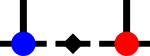SyTen## ◆ write() [1/3]

template<typename Scalar , Rank rank>
 void syten::write ( std::ostream & out, DenseTensor< rank, Scalar > const & t )

Writes a arbitrary-rank dense tensor at the necessary precision to an output stream.

Has the advantage that the inverse operation is also defined for tensors of all ranks: read()

Referenced by syten::Pyten::init_dense_tensor(), and write().Here is the caller graph for this function: